26 Oct 2019

# Introduction

Radar is one of the most versitile sensors we have to determine what is happening in an environment. Some common applications include air traffic control, weather prediction, automated driver assist, astronomy and terrain mapping. Given that these applications have wildy varying requirements on resolution, range and fault tolerance, it’s remarkable that the same fundamental principles can accomodate them all. In this post we will outline a basic blueprint used by most radar implementations and conclude by discussing the modifications and tradeoffs that can be levergaged to improve the performance on a given domain.

The basic operation of a radar involves emmiting radio waves from an antenna, recording the waves that are reflected back, and then analyzing these reflected waves in order to estimate the current state of the environment . In a crowded scene we may simultaneously receive reflections from multiple objects travelling in different directions so picking out the contributions from individual objects presents an interesing signal processing problem.

In the next section we’ll talk about the type of waveform we will be emitting and show how its reflections can be detected with an antenna. After that we’ll analyze what happens when this type of wave is reflected off of a single object with a specified distance and velocity. Then we’ll consider the more general situation in which we simultaneously receive reflections from multiple objects and present a simple algorithm that allows us to pick out individual objects. The algorithm will be based on the fast Fourier transform which was discussed in our previous post on the sampling theorem. Finally, we’ll discuss some of the limitations of our simplified setup and mention some enhancements that are employed by real world systems.

The basic type of signal that we’re interested in emitting is a pulse $A_p(t)$ with a duration of $\tau$ seconds:

$A_p(t) := \begin{cases} 1 & 0 \leq t \leq \tau \\ 0 & \mathrm{else} \end{cases}$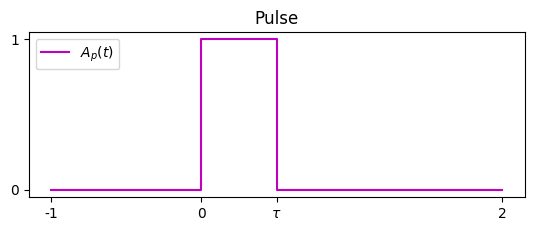The idea is that if $\tau$ is small, then we determine the distance to an object by the time it takes for the pulse to be reflected back.

However, since the signal is being transmitted via a radiowave it can’t actually have a constant amplitude of $1$. Instead, we modulate the signal with a wave $e^{i\Omega t}$ that is oscillating at a fixed frequency $\Omega$ . The resulting modulated signal is:

$\tilde{x}(t) := A_p(t)e^{i\Omega t}$

and the wave $e^{i\Omega t}$ is called a carrier wave.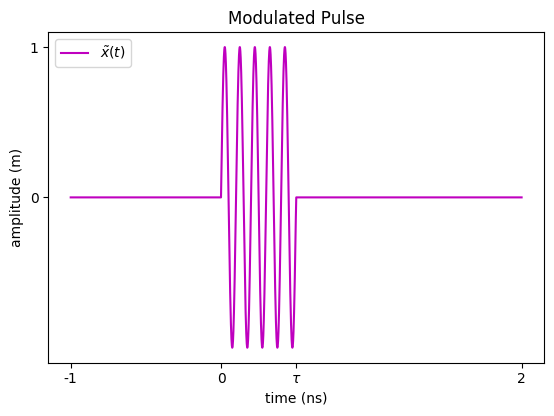If $\tilde{x}(t)$ is reflected off of an object and returns to the radar sensor after $T$ seconds, the sensor will detect the modulated wave:

$\tilde{y}(t) = k A_p(t - T)e^{i(\Omega (t - T) + \theta(t))}$

The constant factor $k$ is less than $1$ and represents that amount of power that has been lost as the signal travelled to the object and back. The shift $t - T$ is due to the fact that we are observing the original wave $\tilde{x}(t)$ after a delay of $T$ seconds. Finally, the addition of the function $\theta(t)$ represents a possible change in the phase of the wave that sometimes occurs as it reflects off an object.

Once we receive $\tilde{y}(t)$, the last step is to demodulate it by effectively multiplying by $e^{-i\Omega (t - T)}$ to recover $y(t)$, the reflection of the original wave $x(t)$:

$y(t) = k A_p(t - T)e^{i \theta(t)}$

The demodulation is done by using a local oscillator with frequency $\Omega$ to maintain the value of $e^{i\Omega t}$. The value of the oscillator is mixed with the received signal to obtain the demodulated form.

You may be wondering how in practice we can transmit and receive the functions $\tilde{x}(t)$ and $\tilde{y}(t)$ since they take complex values. Even though the electromagnetic wave itself is complex, as it has both an electric component $\sin(\Omega t)$ and a magnetic component $\cos(\Omega t)$, an antenna works by detecting the voltage induced by the electric component so we in practice can only measure the real valued signal $kA_p(t - T)\sin(\Omega(t - T) + \theta(t))$. So how is it possible to recover the full complex valued $y(t)$?

We can state the problem more generally as follows: Given the function $\sin(\Omega t + \theta(t))$, how can we recover $e^{i\theta(t)}$? Without knowing anything about $\theta(t)$ this is not possible since for any solution $\theta_1(t)$, we can find another solution $\theta_2(t)$ by demanding that

$\Omega t + \theta_2(t) = -(\Omega t + \theta_1(t)) \Rightarrow \theta_2(t) = -2\Omega t - \theta_1(t)$

In other words, $\theta(t)$ (and therefore also $e^{i\theta(t)}$) is not uniquely detemined by $\sin(\Omega t + \theta(t))$.

Fortunately, in the case of radar we can take advantage of the fact that the absolute value of $\Omega$ is significantly larger than that of $\theta(t)$. This is because the carrier frequency is typically in the hundreds of MHz to GHz range whereas $\theta(t)$, as we will see later, comes from the doppler effect and is roughly on the order of magnitude of the speed of the reflecting object.

This difference allows us to use the following trick. We first multiply $\sin(\Omega t + \theta(t))$ by $2\sin(\Omega t)$ and use standard trig identities to get

$2\sin(\Omega t)\sin(\Omega t + \theta(t)) = \cos(\theta(t)) - \cos(2\Omega t + \theta(t))$

Since $|\Omega t|$ is so much larger than $|\theta(t)|$ we can use a lowpass filter to filter out the high frequency component $\cos(2\Omega t + \theta(t))$ thus recoving the signl $\cos(\theta(t))$.

Similarly, we can also multiply $\sin(\Omega t + \theta(t))$ by $2\cos(\Omega t)$ to get

$2\cos(\Omega t)\sin(\Omega t + \theta(t)) = \sin(\theta(t)) + \sin(2\Omega t + \theta(t))$

and use a lowpass filter to recover $\sin(\theta(t))$. Now that we have both $\cos(\theta(t))$ and $\sin(\theta(t))$ we can of course obtain the full complex signal $e^{i\theta(t)}$ using the Euler identity:

$e^{i\theta(t)} = \cos(\theta(t)) + i\sin(\theta(t))$

Now that we know how it is implemented, for the remainder of this post we can safely assume that we can transmit a complex wave $\tilde{x}(t) = A_p(t)e^{i\Omega t}$ and receive a wave of the form $\tilde{y}(t) = kA_p(t - T)e^{i(\Omega t + \theta(t))}$.

# Single Pulse, Single Object

In this section we’ll see what happens when a single (modulated) pulse $\tilde{x}(t)$ is reflected off of an object back to the radar sensor. In particular, we’ll analyze the wave $\tilde{y}(t)$ received by the sensor and find out how it can be used to determine the distance and speed of the reflecting object.

## The Stationary Case

We’ll start with the easier case in which the object is not moving and is $R$ meters away from the radar. As discussed in the previous section, we emit a modulated pulse at time $t=0$ which means that the transmitted wave has the form

$\tilde{x}(t) = A(t)e^{i\Omega t}$

Since the pulse moves at the speed of light $c$, it will take it $\frac{2R}{c}$ seconds to make the round trip from the sensor to the object and back. So computing the distance $R$ is easy - all we have to do is measure the time $T$ that it takes for the receiver to get a signal and then $R = \frac{cT}{2}$. Even though this is pretty intuitive, it will be helpful to understand this in terms of the received signal $\tilde{y}(t)$ as a warmup for the next section.

In this case, the signal $\tilde{y}(t)$ has the form

$\tilde{y}(t) = kA\left(t - \frac{2R}{c}\right)e^{i\Omega\left(t - \frac{2R}{c}\right)}$

for some constant $0 < k < 1$. As mentioned above, the factor $k$ accounts for the power that is lost during the trip.After demodulation, we get the signal:

$y(t) = kA\left(t - \frac{2R}{c}\right)$

This implies that we can estimate the distance $R$ by measuring the time that it takes for the demodulated received signal to be greater than zero.

## The Constant Velocity Case

We now consider what happens when the pulse is reflected off an object moving with a constant velocity $v$ towards the sensor. Specifically, suppose that the distance of the object as a function of time is $R(t) = R_0 - vt$. To simplify calculations we assume that $v$ is much smaller than the speed of light. As a corollary we will assume that a pulse emitted at time $t$ will take $\frac{2R(t)}{c}$ seconds to return to the sensor. This means that we receive the wave:

\begin{align*} \tilde{y}(t) &= kA\left(t - \frac{2R(t)}{c}\right)e^{i\Omega\left(t - \frac{2R(t)}{c}\right)} \\ &= kA\left(t - \frac{2R_0}{c} + \frac{2vt}{c}\right)e^{i\Omega\left(t - \frac{2R_0}{c} + \frac{2vt}{c}\right)} \\ &\overset{(1)}{=} kA\left(t - \frac{2R_0}{c} + \frac{2vt}{c}\right)e^{i\left(\Omega{t} - \frac{2R_0}{\lambda} + \frac{2vt}{\lambda}\right)} \\ &\overset{(2)}{\simeq} kA\left(t - \frac{2R_0}{c}\right)e^{i\Omega{t}}e^{-i\frac{2R_0}{\lambda}}e^{i\frac{2vt}{\lambda}} \\ &\overset{(3)}{=} k'A\left(t - \frac{2R_0}{c}\right)e^{i\Omega_D{t}}e^{i\Omega{t}} \end{align*}

Equality $(1)$ comes from the identity $c = \frac{\lambda}{\Omega}$ where $\lambda$ being the wavelength of the carrier wave $e^{i\Omega t}$. $(2)$ follows from our assumption that $v \ll c$. Finally, equality $(3)$ is obtained by defining a new constant $k’ := k e^{-i\frac{2R_0}{\lambda}}$ and by defining the doppler frequency to be $\Omega_D := \frac{2v}{\lambda}$. Here is an image with the transmitted modulated signal on top and the received signal on the bottom. Note that the received signal has a lower amplitude and a higher frequency.After demodulation, we get the signal

$y(t) = k’A\left(t - \frac{2R_0}{c}\right)e^{i\Omega_D{t}}$

The goal is now to use this signal to recover the distance $R_0$ and velocity $v$. We’ll start with the distance as it’s quite similar to the stationary case. Note that the absolute value of $y(t)$ is $k’A(t - \frac{2R_0}{c})$ which is zero everywhere except when $t$ is close to $\frac{2R_0}{c}$. This means that as before we can estimate the distance $R_0$ by measuring $|y(t)|$ at multiple points in time and waiting until we get a non zero value. Once we’ve found some $t_R$ such that $|y(t)| > 0$ we can use the identity

$t_R \simeq \frac{2R_0}{c}$

to estimate $R_0$.

Now that we know the distance we can try to estimate the velocity $v$. Recall that the doppler frequency was defined to be $\Omega_D = \frac{2v}{\lambda}$ where $\lambda$ is the frequency of the carrier wave which we already know. So finding $v$ is equivalent to estimating $\Omega_D$. When we estimated the distance we only cared about finding a time $t_R$ for which $|y(t_R)|$ was not zero. In our search for $\Omega_D$ we’ll consider the actual value of the signal $y(t)$ at $t=t_R$ which by the definition of the pulse $A(t)$ is

$y(t_R) = k’e^{i\Omega_D t_R}$

In effect, we’ve managed to obtain a sample of the function $k’e^{i\Omega_D t}$ at time $t=t_R$. Unfortunately, a single sample is not enough to determine the doppler frequency $\Omega_D$. However, recall that if we assume some bound on the magnitude of $\Omega_D$ (i.e the bandwidth) then by the sampling theorem we can recover $\Omega_D$ using multiple samples where the number of samples is determined by the bandwidth. Furthermore, in that post we showed how this can be done efficiently using the fast Fourier transform.

The upshot of this discussion is that even though we cannot measure $\Omega_D$ using a single pulse, we may be able to do it with multiple pulses by using them to obtain many samples of $e^{i\Omega_D t}$ and then applying the FFT. This leads us to the next section in which we’ll upgrade the single pulse to the pulse burst waveform and work through the details of using it to estimate $\Omega_D$.

# The Pulse Burst Waveform

We now introduce the pulse burst waveform and show how to use it to estimate an objects velocity.

A pulse burst is a sequence of $M$ equally spaced pulses where each pulse is emitted $T$ seconds after the beginning of the previous one. We can write this function as

$x(t) = \sum_{m=0}^{M-1} A(t - mT)$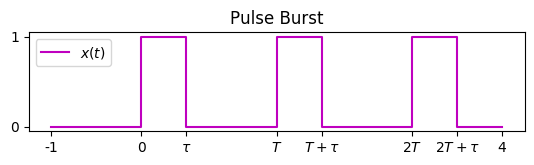As usual, the modulated form is

$\tilde{x}(t) = x(t)e^{2\pi{i}\Omega t} = \sum_{m=0}^{M-1} A(t - mT)e^{2\pi{i}\Omega t}$It’s important to note that even though the pulses are each shifted by $mT$, they are all multiplied by the same carrier wave $e^{2\pi{i}\Omega t}$. For this reason we call the pulses coherent.

## Detecting a Single Object

Suppose that the pulse burst reflects off of an object at distance $R_0$ meters moving towards the sensor at $v$ meters per second. Since each pulse comes back to the sensor after $\frac{2R_0}{c}$ seconds, we can repeat the same calculation as we did for the single pulse waveform to conclude that the received (demodulated) signal will be

$y(t) = \sum_{m=0}^{M-1}k A\left(t - mT - \frac{2R_0}{c}\right)e^{\Omega_D t}$

where $\Omega_D = \frac{2v}{\lambda}$ and $k$ is a constant.

We’ll now see how to estimate the distance $R_0$ and velocity $v$ by sampling $y(t)$. After each pulse, we measure $L$ samples spaced by $T_s$ seconds for a total for $L \times M$ samples: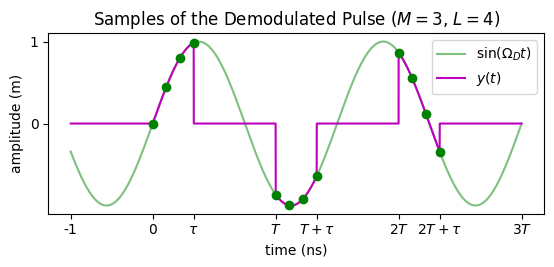We collect these samples in an $L$ by $M$ grid $y[l, m]$ by putting the result of the $l$-th sample following the $m$-th pulse in cell $y[l, m]$. By definition, the $y[l, m]$ contains the value of $y(t)$ at $t = mT + lT_s$ so

\begin{align*} y[l, m] = y(mT + lT_s) &= \sum_{k=0}^{M-1}k A\left(mT + lT_s - kT - \frac{2R_0}{c}\right)e^{i\Omega_D (mT + lT_s)} \\ &= k A\left(lT_s - \frac{2R_0}{c}\right)e^{i\Omega_D (mT + lT_s)} \\ &= \begin{cases} k e^{i\Omega_D (mT + lT_s)} & lT_s \simeq \frac{2R_0}{c} \\ 0 & \mathrm{else} \end{cases} \end{align*}

where the last equality follows from the definition of the pulse $A(t)$. In conclusion, all of the rows of $y[l, m]$ will be $0$ except for row $l=l_0$ satisfying $l_0 \simeq \frac{2R_0}{cT_s}$. To simplify notation, we define $W_D := e^{i\Omega_D T}$. We can now draw the contents of the data grid up to a scalar as: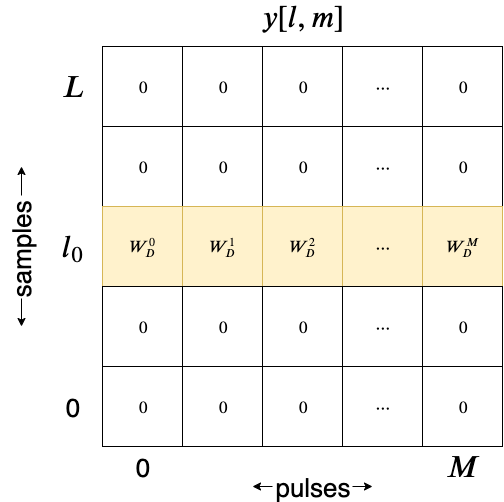This already allows us to estimate the distance by finding the non zero row $l_0$ and concluding that

$R_0 \simeq \frac{l_0 cT_s}{2}$

We now turn to estimating the velocity $v$ or equivalently, the doppler frequency $\Omega_D$. To do this we consider the $l_0$-th row of the data grid:

$y[l_0, m] = k e^{i\Omega_D (mT + l_0T_s)} = k’ e^{i\Omega_D mT}$

where we’ve absorbed the constant $e^{i\Omega_D l_0T_s}$ into the leading constant $k$. We can see that values in row $l_0$ consist of $M$ equally spaced samples of the function $f(t) = k’ e^{i\Omega_D t}$ on the interval $[0, MT)$. Explicitly, we have sampled $f(t)$ at the points $t = 0, T, \dots, (M-1)T$ and got length $M$ vector $\mathbf{v} = [y[l_0, 0], \dots, y[l_0, M-1]]$. As we explained in our sampling theorem post, if we apply fast Fourier transform to $\mathbf{v}$ then the result $\mathbf{w} = \mathrm{FFT}(\mathbf{v})$ will also be a length $M$ vector satisfying

$f(t) = \sum_{k=0}^{M-1}w_k e^{2\pi{i}\frac{k}{MT}t}$

In this case, since by definition $f(t)$ has a single Fourier component $\Omega_D$, the vector $\mathbf{w}$ will be zero everywhere except for the index $k=k_0$ satisfying

$\Omega_D \simeq \frac{2\pi k_0}{MT}$

Since the rest of the rows of the data grid $y[l, m]$ are zero, this means that if we apply the FFT to each row of $y[l, m]$ we’ll get a grid $z[l, k]$ with only a single non zero element at $z[l_0, k_0]$. We call $z[l, k]$ the Fourier grid.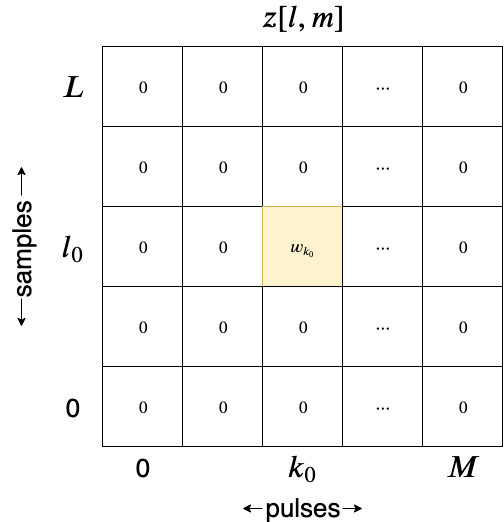It is now clear how to estimate the distance $R_0$ and velocity $v$. We send out a pulse burst, sample the returns and record them in the data grid $y[l, m]$. We then apply a FFT to each row of $y[l, m]$ and get the Fourier grid $z[l, k]$. Next we find the single non-zero cell $(l_0, k_0)$ in $z[l, k]$. Our estimate for the distance is then

$R_0 \simeq l_0 \frac{cT_s}{2}$

and our estimate for the doppler frequency is $\Omega_D \simeq k_0\frac{2\pi}{MT}$. Since $\Omega_D = \frac{2v}{\lambda}$, the velocity may be approximated by

$v \simeq k_0 \frac{\pi\lambda}{MT}$

## Detecting Multiple Objects

We now consider what happens when the pulse burst reflects off of $N$ objects. In this case, the received signal will simply be a sum of the signal coming from each object. Specifically, this means that the data grid $y[l, m]$ will be a sum of grids $y_i[l, m]$, where $y_i[l, m]$ is the grid corresponding to the $i$-th object as described in the previous section. Since the FFT is a linear transformation, we get the Fourier grid

\begin{align*} z[l, k] &= FFT(y[l, m]) = FFT(\sum_{i=0}^{N-1} y_i[l, m]) \\ &= \sum_{i=0}^{N-1} FFT(y_i[l, m]) = \sum_{i=0}^{N-1} z_i[l, k] \end{align*}

As we saw in the previous section, the Fourier grid $z_i[l, k]$ has zeros everywhere except for at a single cell representing the distance and velocity of the $i$-th object. Therefore, since $z[l, k] = \sum_{i=0}^{N-1} z_i[l, k]$, $z[l, k]$ will have zeros everywhere except for $N$ cells, one cell per object.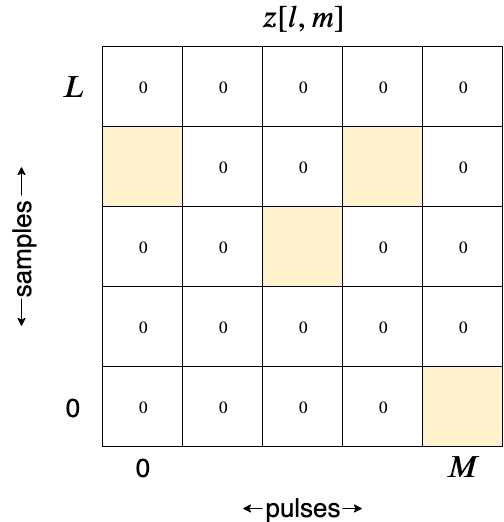For each non-zero cell $(l_i, k_i)$ we estimate an object with distance

$R_i \simeq l_i \frac{cT_s}{2}$

and velocity

$v_i \simeq k_i \frac{\pi\lambda}{MT}$

The resulting algorithm for the case of multiple objects is quite simple - we send out a pulse burst, sample the returned signal in the data grid $y[l, m]$, apply the FFT to each row to get the Fourier grid $z[l, k]$ and estimate a distance and velocity for each non-zero element of $z[l, k]$.

# Limitations and Ideas for Improvement

The algorithm we presented in the previous section is a bit too simple and in fact we’ve swept a couple of key difficulies under the rug. Dealing with these difficulties makes up the majority of work involved in designing a robust system. Furthermore, as we will see, overcoming these issues presents us with tradeoffs such as choosing between a high resolution in range and a high resolution in velocity. These inherent tradeoffs are the reason that it is not possible to design a single radar system that will perform well in all of the applications listed in the introduction. For instance, a weather radar will have a long range, but poor range resolution makeing it unsuitable for cruise control on a car.

## The Signal to Noise Ratio

In any electronic system there are sources of noise such as thermal noise in which variations in temperature give rise to small changes in important quantaties such as the resistance of certain materials. Another source of noise is the environment itself which in practice will not have the exact properties we used in the derivation of our algorithm. In order to get good estimate from the radar system, the values in the data grid $y[l, m]$ must be greater, and if possible significantly greater, than the expected amount of noise. The ratio between the expected values in the grid and the expected amount of noise is called the signal to noise ratio and is typically denoted by $\chi$.

We can in fact make a more quantitative statement about the significance of $\chi$. As we discussed in this previous post, for any estimator of a variable $x$ based on a noisy signal $y$ there is a fundamental quantity called the Cramer-Rao lower bound which gives us a lower bound on the expected size of the error that we will make when estimating $x$. We can view the radar system as an estimator of positions and velocities based on noisy observations of the reflections of the pulse burst. It turns out the Cramer-Rao lower bound of this radar estimator is inversely proportional to $\chi$. So if we increase the signal to noise ratio by a factor of two, we will half the expected amount of error in our estimates.

Increasing the signal to noise ratio is a goal which influences everything from chip design to the details of the signal processing implementation.

As an example, it turns out that the pulse burst we used is not very good from a signal to noise perspective. The issue is that since the signal is constant throughout the duration of a pulse, we loose energy from the points in time that were never sampled. One way to fix this is known as matched filtering which aims to concentrate the signal $y(t)$ around the points at which we are sampling. In general, there is a vast literature concerned with the signal to noise properties of different types of wave forms and signal processing techniques.

## Max Distance vs Max Velocity

Let’s take a closer look at the maximum distance and velocity we can meausre using the radar we described. Starting with the distance measurment, note that if a reflection of pulse $m$ returns to the sensor after pulse $m+1$ has been fired, then we have no way of knowing which of the two pulses the reflection came from. As we’ve seen, if an object is at distance $R$ then it will take $\frac{2R}{c}$ seconds for the reflection to return. Since the time between consecutive pulses is $T$, this maximum distance we can measure satisfies

$\frac{2R_{\mathrm{max}}}{c} = T \Rightarrow R_{\mathrm{max}} = \frac{cT}{2}$

What about the maximum velocity? Recall that we calculate velocities by finding a non zero cell $z[l_0, k_0]$ and estimating the velocity as

$v \simeq k_0 \frac{\pi\lambda}{MT}$

Since $0 \leq k \leq M-1$, this means that the maximum velocity we can measure is

$v_{\mathrm{max}} = \frac{(M-1)\pi\lambda}{MT} \simeq \frac{\pi\lambda}{T}$

So we see that the maximum distance we can measure is proportional to $T$ whereas the maximum velocity we can measure is inversely proportional to $T$. This means that with the pulse burst design we can either detect far objects or fast objects but not both. One way to work around this problem is to use a non uniform spaceing of the pulses which is known as staggering. However, this greatly complicates the signal processing necessary to extract velocities.

## Range Resolution vs Velocity Resolution

We now take a look at the resolution of our algorithm in both range and velocity. Recall that we estimate range based on the time it takes for a pulse to return. However, the pulse itself has duration which we denoted $\tau$. When we receive a signal, we have no way of knowing whether it comes from the reflection of the beginning or end of the pulse. This ambiguity of $\tau$ seconds translates to an ambiguity of $c \tau$ meters in our range estimate. In other words, the resolution of our range estimate is $c \tau$ meters.

On the other hand, we estimate the velocity by essentially sampling from a Fourier transform of the pulse. A simple calculation shows that the Fourier transform of a short pulse is wide whereas the transform of a long pulse is narrow. In other words, the “width” of the Fourier transform of a pulse is inversely proportional to the duration of the pulse. This implies that the resolution of our velocity estimate is inversely proportional to the pulse duration $\tau$.

So we have another tradeoff to make between having good range resolution and good velocity resolution. One way to circumvent this is to replace the simple pulse with what is known as a chirp. A chirp is similar to a pulse, except that rather than having a constant frequency of $0$ the frequency starts at $0$ and increases at a fixed rate $\beta$ hz per second. The name “chirp” comes from the sound that you’d get from a soundwave with this form. The rate of increase in frequency $\beta$ gives us another variable to play with in the design of the pulse which can allow us to achieve high resolution in both range and velocity.

## Azimuth Estimation

In most practical cases we are interested in measuring not only the objects range but also its angle $\theta$ with respect the x-axis: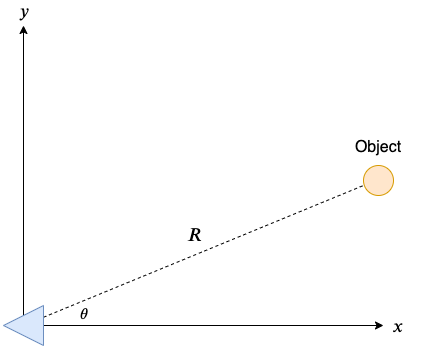Interestingly, we can estimate $\theta$ using the same type of algorithm that we used to measure velocity. To do this, we will replace our single receiver with an array of $2N + 1$ sensors placed $d$ meters apart along the $y$ axis and centered at the origin. Sensor $n$ will have a y coordinate of $nd$ for $-N \leq n \leq N$. Here is an example with $N=1$:The dotted lines in the image represent rays coming from the object and hitting the sensors. As we can see from the diagram, the ray hitting the sensor immediately below the origin ($n=-1$) will arrive with a delay of $\frac{d\sin(\theta)}{c}$ seconds. In other words, if the sensor at the origin receives the modulated signal

$\tilde{y}_0(t) = k’A(t - \frac{2R}{c})e^{i\Omega t}$

then the sensor below it will receive the modulated signal

\begin{align*} \tilde{y}_{-1}(t) &= k'A(t + \frac{d\sin(\theta)}{c} - \frac{2R}{c})e^{i\Omega (t + \frac{d\sin(\theta)}{c})} \\ &\simeq k'A\left(t - \frac{2R}{c}\right)e^{i\Omega t}e^{i \frac{\sin(\theta)}{\lambda} d} \end{align*}

In the second approximation we used the fact that $\frac{d\sin(\theta)}{c}$ is very small as well as the identity $\Omega = \frac{c}{\lambda}$. The demodulated signal is thus:

\begin{align*} y_{-1}(t) \simeq k'A\left(t - \frac{2R}{c}\right)e^{i \frac{\sin(\theta)}{\lambda} d} \end{align*}

As before, by the definition of the pulse $A(t)$ this signal is equal to zero except when $t \simeq t_R$ where $t_R := \frac{2R}{c}$. At that time, we get the signal

$y_{-1}(t_R) \simeq k’ e^{i \frac{\sin(\theta)}{\lambda} d}$

Generalizing this to the $n$-th sensor, it is not hard to see that

$y_{n}(t_R) \simeq k’ e^{i \frac{\sin(\theta)}{\lambda} -nd}$

for $-N \leq n \leq N$. Lets collect these measurements into a vector $\mathbf{v} := [y_{-N}(t_R), \dots, y_{N}(t_R)]$. It is clear that $\mathbf{v}$ contains $2N+1$ evenly spaced samples of the function $f(t) = e^{i \frac{\sin(\theta)}{\lambda} t}$ in the interval $[-Nd, Nd]$. Therefore, similarly to what we did to compute the velocity, we can compute $\frac{\sin(\theta)}{\lambda}$ by applying the FFT to $\mathbf{v}$, and from there it is easy to compute the azimuth $\theta$.